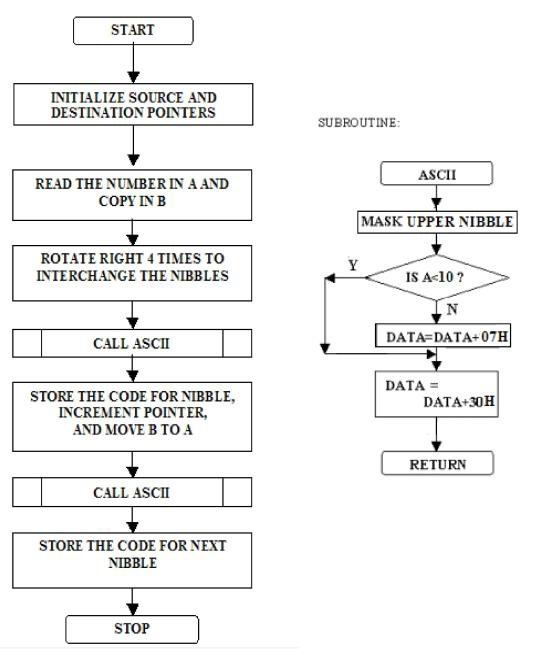# Program to convert two-digit hex to two ASCII values in 8085 Microprocessor

8085MicrocontrollerMicroprocessor

Here we will see a program of Intel 8085 Microprocessor. Using this program, we can convert 8-bit numbers to two digit ASCII values.

Problem Statement

Write an 8085 Assembly language program to convert 8-bit binary to 2-character ASCII values. The 8-bit binary number is stored in memory location 8050H. Separate each nibbles and convert it to corresponding ASCII code and store it to the memory location 8060H and 8061H.

Discussion

In this problem we are using a subroutine to convert one hexadecimal digit (nibble) to its equivalent ASCII values. As the 8-bit number contains two nibbles, so we can execute this subroutine to find ASCII values of them. We can get the lower nibble very easily by masking the upper nibble, and for the upper nibble, we have to mask the lower nibble at first, then rotate the register content dour times to the right to make, now we can change it to ASCII values.

Here we will put 3AH as input, the program will return 33 and 41. These are the ASCII values of 3 and A respectively.

Data

8050
3A

## Flow Diagram## Program

HEX Codes
Labels
Mnemonics
8000
31, 00, 81

LXI SP, 8100
Initialize SP
8003
21, 50, 80
START
LXI H, 8050H
Initialize pointer with the first location of IN-BUFFER
8006
11, 60, 80

LXI D, 8060H
Initialize pointer with the first location of OUT-BUFFER
8009
7E

MOV A, M
Move the contents of 8050H to A
800A
47

MOV B, A
Copy A to B
800B
0F

RRC
Rotate accumulator right 4 times
800C
0F

RRC

800D
0F

RRC

800E
0F

RRC

800F
CD, 1A, 80

CALL ASCII
This subroutine converts a binary no. to ASCII
8012
12

STAX D
Store the contents of the accumulator specified the contents by DE register pair
8013
13

INX D
Go to next location
8014
78

MOV A, B
Copy B to A
8015
CD, 1A, 80

CALL ASCII
This subroutine converts a binary no. to ASCII
8018
12

STAX D
Store the contents of the accumulator specified the contents by DE register pair
8019
76

HLT
Terminate the program
801A
E6, 0F
ASCII
ANI 0FH
Converts a BCD number to its corresponding
ASCII value
+ 48
0 To 9 -----------------à 48 To 57
+ 55
A To F -----------------à 65 To 70
+ 48 + 7
So +48 is common but if the hex digit is
between A to F then +7 is additional.
801C
FE, 0A

CPI 0AH

801E
DA, 23, 80

JC CODE

8021
C6, 07

8023
C6, 30
CODE

8025
C9

RET
Returning control to the calling program